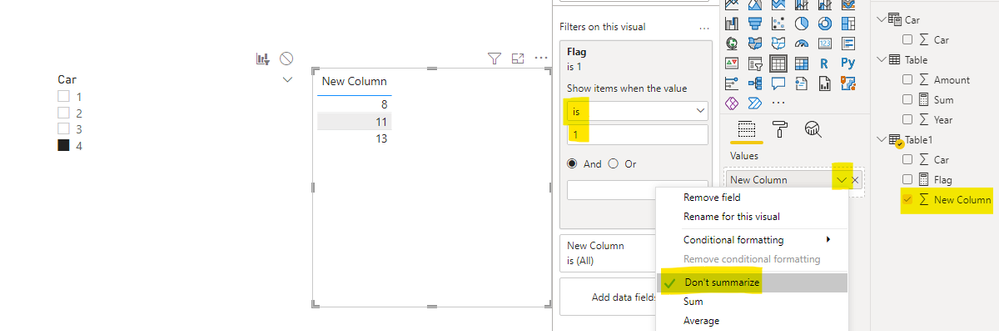cancel
Showing results for
Did you mean:New Member

## Sum If - Same Column

Hi all,

I am having a problem to solved this problem:

I want to create a sumif that references a cell to the whole column.
Example below:

I have a column with cars and I would like to create a column that sums all the lines that the number is more than the referent column.

 Car New Column 1 13 2 11 3 8 4 0 4 0

In excel it would be something like =SUMIF(B8:B12,">"&B8), but in DAX I don't know if it's possible to solve.
In my view would below line, but it is not working

CALCULATE(SUM(Data[Car]),Data[Car]>Data[Car])

Regards,

1 ACCEPTED SOLUTIONCommunity Support

Hi, @I1qq6uyz

According to your description, you want to create a column that shows all the lines that the number is more than the referent column, right?

In the Power BI, you can just use the table chart to display as a new column because this column is filtered by the selection of a slicer. I think you can create a new table and a slicer to filter a table chart to achieve your requirement, you can follow my steps:

Create a calculated table:

``Car = SUMMARIZE('Table1',Table1[Car])``

Create a measure like this:

``````Flag =

var _selectedcolumn=CALCULATE(MAX('Table1'[New Column]),FILTER(ALL('Table1'),[Car]=SELECTEDVALUE('Car'[Car])))

return

IF(MAX('Table1'[New Column])>_selectedcolumn,1,0)``````

Then you can create a car slicer to place the column in the new table and a table chart to set the filter and summarize type like this:Thank you very much!

Best Regards,

Community Support Team _Robert Qin

If this post helps, then please consider Accept it as the solution to help the other members find it more quickly.

2 REPLIES 2Community Support

Hi, @I1qq6uyz

According to your description, you want to create a column that shows all the lines that the number is more than the referent column, right?

In the Power BI, you can just use the table chart to display as a new column because this column is filtered by the selection of a slicer. I think you can create a new table and a slicer to filter a table chart to achieve your requirement, you can follow my steps:

Create a calculated table:

``Car = SUMMARIZE('Table1',Table1[Car])``

Create a measure like this:

``````Flag =

var _selectedcolumn=CALCULATE(MAX('Table1'[New Column]),FILTER(ALL('Table1'),[Car]=SELECTEDVALUE('Car'[Car])))

return

IF(MAX('Table1'[New Column])>_selectedcolumn,1,0)``````

Then you can create a car slicer to place the column in the new table and a table chart to set the filter and summarize type like this:Thank you very much!

Best Regards,

Community Support Team _Robert Qin

If this post helps, then please consider Accept it as the solution to help the other members find it more quickly.Super User

Hello there @I1qq6uyz ! Not quite sure if I got your question correctly but try this:

``CALCULATE(SUM(Data[Car]),Data[Car]>EARLIER(Data[Car]))``

If my reply provided you with a solution, please consider marking it as a solution ✔️ or giving it a kudoe 👍
Thanks!

You can also check out my LinkedIn!

Best regards,
Gonçalo Geraldes